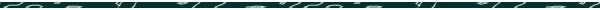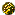Bible Prophecy Year
of 360 Days

Overview of the 1000-year Calendar
and the Eternal Calendar

The following two tables briefly examines these twin calendars.

The Six Cycles of "The 1000-year Calendar"

 One year (360 days) x 40 = 40-year cycle a normal leap month (390 days) x 100 = 4000-year cycle the normal leap month omitted (360 days) x 24 = 96,000-year cycle the normal leap month omitted twice (360 days twice) x 150 = 14,400,000-year cycle the normal leap month omitted (360 days) x 24 = 345,600,000-year cycle a normal leap month (390 days)

The 1000-year Calendar:
Normal and Symbolic Years

 Normal Years Symbolic years: 1000 years = 1 day One year = .0001 days x 40 = 40-year cycle = .04 days (.96 hours) x 100 = 4000-year cycle = 4 days x 24 = 96,000-year cycle = 96 days x 150 = 14,400,000-year cycle = 40 years x 24 = 345,600,000-year cycle = 960 years

1a) The 1000-years-as-One-Day Calendar (1Pet. 3:8)

The solar year as 365.242189670139 days
(Or to be exact...365.2421896701388888888888...)There are 360,000 days in 1000 years, (1000 times a prophetic year of 360 days = 360,000 days). Thus, 360,000 days (i.e., 1000 years) symbolically equals one day.

The broadening cycles of 1 x 40 x 100 x 24 x 150 x 24 ( = 345,600,000-years), viewed as...

40 (x 100 x 24 x 150) x 24 years ==> 40 (x 360,000) x 24 years.

Because the middle cycles of 100 x 24 x 150 equals 360,000, therefore 40 (x 360,000 literal years) x 24 is as, 40 (x 1 symbolic "year") x 24 = 960 symbolic "years;"

or, leaving out the last cycle would mean: 40 (x 1 ) = 40 symbolic "years".(To convert to "days," simply multiply all the above by 360 days.)

 Note: Both "40" and "960" as literal years are a fundamental part of the Prophetic Calendar. Thus the Prophetic Calendar essential ends by repeating its beginning, only now on the 1000-years-equals-one-day ratio!

Here is another way of looking at it:

1 year x 40 x 100 = the 4000-year cycle, which is the same as 4 days on the thousand-years-as-one-day scale.

Therefore, "4 days" times the next broadening cycle of 24x equals as 96 days;

"96 days" times the next broadening cycle of 150x equals as 14,400 days; and 14,400 days = as 40 years (of 360 days).

"14,400 days" times the next broadening cycle of 24x equals as 345,600 days; And  345,600 days = as 960 years (of 360 days.)

Observe that the first cycle of 40 (literal) years on the thousand-years-as-one-day scale symbolically equals as .04 "days", that is, .96 "hours," or 3,456 "seconds." Compare these symbolic days, hours, and seconds with the above figures in red, which leads us to the following astonishing fact:

Not only is "1000-years like a day," but the reverse ratio is also true: "A day is as a 1000 years!"

1b) "One-Day-is-as-a-Thousand-Years" (1Pet. 3:8)

Briefly, there are 3,600 seconds in an hour (60 x 60), and 24 hours in a day.

Therefore, 3,600 sec. x 24 hr. ( = 1 day) x 360 days = 1 prophetic year:

Therefore, we may rewrite the series of cycles as follows: (Note the color coding.)

(3,600 sec. x 24 hrs) x (360 days [ = 1 year.], x the broadening cycles of  40 x 100) x (24 x 150 x 24) seconds;

or simply...

(3,600 x 24) x (360 x 40 x 100) x (24 x 3,600) seconds.

The first bracket "(3,600 x 24)" composes one literal day (of 86,400 seconds);

The middle bracket "(360 d. x 40 x 100)" = 1,440,000 literal days, and is but 4 figurative "days" on the ratio of "A thousand years is as a day."

The last bracket "(24 x 3,600)" signifies a reversal of the first bracket suggesting a figurative return to seconds---hence the reverse ratio of "A day is as a thousand years."

(3,600 x 24) x (360 x 40 x 100) x (24 x 3,600)
==> ==>   ~~~~~~~~~~~   <==  <==

Or another way of looking at it:

There are 86,400 seconds in a day (60 x 60 x 24), and the last three cycles also add up to a value of 86,400 (24 x 150  x 24). The middle figures of "360 days x 40" = 14,400 days, or 40 years, which is one generation. Therefore:

86,400 seconds ==> times one generation <==  times 86,400 seconds

 Furthermore, the first three cycles of 360 days x 40 x 100 are 100 over 6 days (or, 16.666...) that of the last three cycles of 24 x 150 x 24. (In the bible numbers, "1666" is consistently a transfer/switch number. To be explained at another time.)The Eternal Calendar simply continues from where the 1000-year Calendar ended.

Perpetual Cycles of "The Eternal Calendar"

 One year (360 days) x 40 = 40-year cycle a normal leap month (390 days) x 100 = 4000-year cycle the normal leap month omitted (360 days) x 24 = 96,000-year cycle the normal leap month omitted twice (360 days twice) x 150 = 14,400,000-year cycle the normal leap month omitted (360 days) x 24 = 345,600,000-year cycle a normal leap month (390 days) x 150 = 51,840,000,000-year cycle the normal leap month omitted (360 days) x 24 = 1,244,160,000,000-year cycle the normal leap month omitted twice (360 days twice) x 150 = 186,624,000,000,000-year cycle the normal leap month omitted (360 days) x 24 = 4,478,976,000,000,000-year cycle, etc... a normal leap month (390 days)

The Eternal Calendar:
Normal and Symbolic Years

 Normal Years Symbolic years: 1000 years = 1 day One year = .0001 days x 40 = 40-year cycle = .04 days (.96 hours) x 100 = 4000-year cycle = 4 days x 24 = 96,000-year cycle = 96 days x 150 = 14,400,000-year cycle = 40 years x 24 = 345,600,000-year cycle = 960 years x 150 = 51,840,000,000-year cycle = 144000 years (again ÷ 360,000 = .4) x 24 = 1,244,160,000,000-year cycle = 3,456,000 years (again ÷ 360,000 = 9.6) x 150 = 186,624000,000,000-year cycle = 518,400,000 years (again ÷ 360,000 twice = 4) x 24 = 4,478,976,000,000,000-year cycle, = 12,441,600,000 years (again ÷ 360,000 twice = 96) etc... etc...

 2) The Eternal Calendar    The solar year as 365.24218966953624... daysThe broadening cycles are themselves grouped in cycles of four. That is, every fourth broadening cycle repeats the same form of leap-month adjustment. For example, the 40-year cycle is "normal," in that a leap-month is added as normal. And likewise, four broadening cycles later (i.e. 40 x 100 x 24 x 150 x 24) the leap month is again added normally. Thus, the solar year of 365.242189670139 of the "1000-year Calendar" creates one fourfold broadening cycle, but no more. This implies that the calendar is meant to persist onto yet larger cycles, and clusters of broadening cycles; hence, "The Eternal Calendar." Furthermore, the 40 x 100 x 24 x 150 x 24 broadening cycles of the 1000-year calendar suggests that the Eternal Calendar should look like so: 1 x 40 x 100 x 24 x 150 x 24 x 150 x 24 x 150 x 24 x 150… The 24 x 150 x 24 x 150 pattern… forms interlocking multiples of 3,600, (i.e., 24 x 150 = 3,600, as does the reverse of 150 x 24, etc.) Therefore, each fourfold broadening cycle would be 3,600 squared, (or, 60 x 60 x 60). (See "Square Root and the Prophetic Calendar.")    (See "Evidence for the Eternal Calendar" for a fuller treatment  of why the 1000-year calendar can be perpetuated.)

Also see Bible Numbers

New-Years Day on the 360 Calendar